# KR101621244B1 - Counter Circuit, Device Including the Same, and Counting Method - Google Patents

## Info

Publication number
KR101621244B1
KR101621244B1 KR1020090011692A KR20090011692A KR101621244B1 KR 101621244 B1 KR101621244 B1 KR 101621244B1 KR 1020090011692 A KR1020090011692 A KR 1020090011692A KR 20090011692 A KR20090011692 A KR 20090011692A KR 101621244 B1 KR101621244 B1 KR 101621244B1
Authority
KR
South Korea
Prior art keywords
signal
counting
response
bit
input clock
Prior art date
Application number
KR1020090011692A
Other languages
Korean (ko)
Other versions
KR20100092542A (en
Inventor
임용
고경민
김경민
Original Assignee
삼성전자주식회사
Priority date (The priority date is an assumption and is not a legal conclusion. Google has not performed a legal analysis and makes no representation as to the accuracy of the date listed.)
Filing date
Publication date
Application filed by 삼성전자주식회사 filed Critical 삼성전자주식회사
Priority to KR1020090011692A priority Critical patent/KR101621244B1/en
Publication of KR20100092542A publication Critical patent/KR20100092542A/en
Application granted granted Critical
Publication of KR101621244B1 publication Critical patent/KR101621244B1/en

## Images

•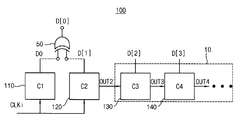•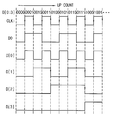•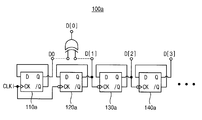•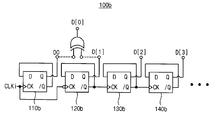•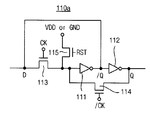•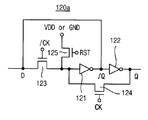••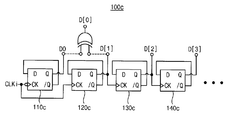•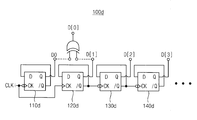••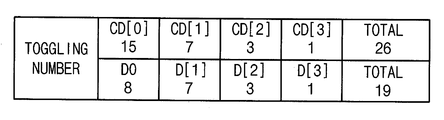•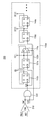•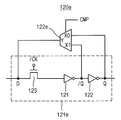•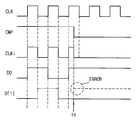•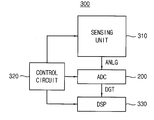•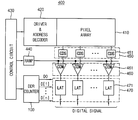•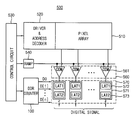•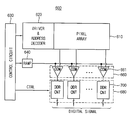•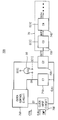•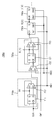••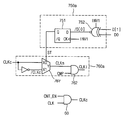•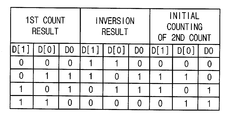•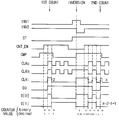•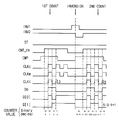•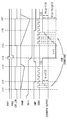•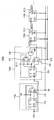•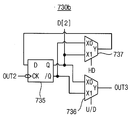•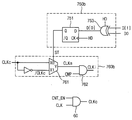•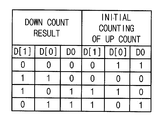•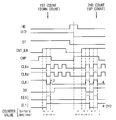•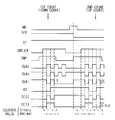•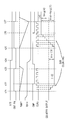•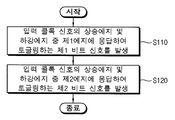•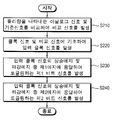•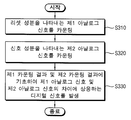## Classifications

• HELECTRICITY
• H03BASIC ELECTRONIC CIRCUITRY
• H03KPULSE TECHNIQUE
• H03K21/00Details of pulse counters or frequency dividers
• H03K21/02Input circuits
• H03K21/023Input circuits comprising pulse shaping or differentiating circuits
• HELECTRICITY
• H03BASIC ELECTRONIC CIRCUITRY
• H03KPULSE TECHNIQUE
• H03K21/00Details of pulse counters or frequency dividers
• H03K21/38Starting, stopping or resetting the counter
• HELECTRICITY
• H03BASIC ELECTRONIC CIRCUITRY
• H03KPULSE TECHNIQUE
• H03K23/00Pulse counters comprising counting chains; Frequency dividers comprising counting chains
• H03K23/40Gating or clocking signals applied to all stages, i.e. synchronous counters
• H03K23/50Gating or clocking signals applied to all stages, i.e. synchronous counters using bi-stable regenerative trigger circuits
• H03K23/54Ring counters, i.e. feedback shift register counters
• H03K23/548Reversible counters

## Abstract

The counter circuit includes a first counting unit and a second counting unit. The first counting unit generates a first bit signal that toggles in response to a first one of a rising edge and a falling edge of the input clock signal and the second counting unit generates a rising edge and a falling edge of the input clock signal And generates a second bit signal that toggles in response to the second edge. The counter circuit performs counting twice every cycle period of the input clock signal to have an improved operating speed and operation margin and reduce the number of toggling of bit signals to reduce power consumption.

## Description

BACKGROUND OF THE INVENTION 1. Field of the Invention The present invention relates to a counter circuit,

BACKGROUND OF THE INVENTION 1. Field of the Invention The present invention relates to counting using a clock signal, and more particularly, to a double data rate (DDR) counter circuit capable of performing a counting operation efficiently, an apparatus including the counter circuit, and a counting method.

A counter circuit can be used in various electronic devices to convert effective physical quantities such as intensity of light, intensity of sound, time, etc. to digital signals.

For example, an image sensor is an apparatus for acquiring an image using the property of a semiconductor that reacts with incident light, and includes an analog-to-digital converter for converting an analog signal output from the pixel array into a digital signal. The analog-to-digital converter may be implemented using a counter circuit that performs a counting operation using a clock signal.

The operating speed and power consumption of the counter circuit have a direct impact on the performance of the device or system comprising it. In particular, the CMOS image sensor may include a plurality of counter circuits for converting the analog signals output from the active pixel sensor array into digital signals according to the configuration thereof. The number of the counter circuits increases according to the resolution of the image sensor. As the number of the counter circuits increases, the operation speed and power consumption of the counter circuit can be an important factor for determining the overall performance of the image sensor.

An object of the present invention is to provide a counter circuit and a counting method capable of reducing power consumption and increasing an operating speed.

It is an object of the present invention to provide an analog-to-digital converter and an analog-to-digital conversion method which can reduce the consumed power and increase the operation speed by using the counter circuit.

It is an object of the present invention to provide an apparatus and a correlated double sampling method which can reduce consumption power and increase operation speed by using the counter circuit.

To achieve the above object, a counter circuit according to an embodiment of the present invention includes a first counting unit and a second counting unit. The first counting unit generates a first bit signal that toggles in response to a first one of a rising edge and a falling edge of the input clock signal. The second counting unit generates a second bit signal that toggles in response to a second one of a rising edge and a falling edge of the input clock signal.

In one embodiment, the counter circuit may further include a ripple counter for generating high bit signals that toggle in response to the second bit signal or the inverted signal of the second bit signal.

Wherein the first counting unit generates the first bit signal in response to a first one of a rising edge and a falling edge of the input clock signal and the second counting unit is responsive to a rising edge and a falling edge of the input clock signal, 2 &lt; / RTI &gt; edge.

The counter circuit may perform a counting operation twice per cycle period of the input clock signal.

In one embodiment, the counter circuit may further comprise a code converter for generating a least significant bit signal of the binary code based on the first bit signal and the second bit signal.

Wherein the first counting unit, the second counting unit and the ripple counter comprise a plurality of D-flip flops each outputting the first bit signal, the second bit signal and the upper bit signals, The first D-flip flop of the unit and the second D-flip flop of the second counting unit may complementarily toggle with respect to the rising or falling edge of the input clock signal.

In one embodiment, the first counting unit includes a rising edge triggered D-flip flop, the second counting unit includes a falling edge triggered D-flip flop, and the counter circuit performs an up- can do.

In another embodiment, the first counting unit includes a falling edge triggered D-flip flop, the second counting unit includes a rising edge triggered D-flip flop, and the counter circuit performs a down counting operation can do.

In one embodiment, the second counting unit may include a feedback switch for interrupting the toggling of the second bit signal in response to a comparison signal indicative of an end time of the counting operation.

In one embodiment, the counter circuit comprises: a clock control circuit for generating a clock control signal based on the first bit signal and the second bit signal; and a clock input for inverting the input clock signal in response to the clock control signal Circuit. &Lt; / RTI &gt;

Wherein the clock control circuit comprises: a logic gate for logically calculating and outputting the first bit signal and the second bit signal; and a control circuit for outputting the clock signal in response to a control signal applied to an output of the logic gate and a clock terminal applied to a data terminal, And a D-flip-flop for outputting a control signal.

Wherein the clock input circuit comprises a multiplexer for selecting and outputting a clock signal or an inverted clock signal in response to the clock control signal and a comparator for comparing the output signal of the multiplexer and the ending time of the counting operation, And outputting the logical product gate.

In one embodiment, the counter circuit may further include an inversion control section for inverting the second bit signal and the higher bit signals in response to the inversion control signal.

The inversion control unit may include a plurality of multiplexers for selecting and outputting one of a front output signal and a second inversion control signal in response to the first inversion control signal.

In one embodiment, the counter circuit may further include a mode switching control section for controlling an up-counting operation or a down-counting operation of the counter circuit in response to a mode control signal.

The mode switching control unit may include a plurality of multiplexers for selecting either one of the non-inverted output terminal of the previous stage or the inverted output terminal of the previous stage in response to the mode control signal and outputting the selected signal to the subsequent stage.

To achieve the above object, an analog-to-digital converter includes a comparator, a clock input circuit, and a counter circuit. The comparator compares an analog signal representing a physical quantity and a reference signal to generate a comparison signal. The clock input circuit generates an input clock signal based on the clock signal and the comparison signal. The counter circuit counts the input clock signal to generate a digital signal corresponding to the analog signal. Wherein the counter circuit includes a first counting unit for generating a first bit signal that toggles in response to a first one of a rising edge and a falling edge of the input clock signal and a second counting unit for producing a rising edge and a falling edge of the input clock signal, And a second counting unit for generating a second bit signal toggling in response to the second edge.

In one embodiment, the counter circuit may further comprise a ripple counter for generating high bit signals that toggle in response to the second bit signal or the inverted signal of the second bit signal

The second counting unit further includes a feedback switch for selectively connecting the inverted output terminal or the non-inverted output terminal of the second D flip-flop to the data terminal of the second D flip-flop in response to the comparison signal can do.

To achieve the above object, an apparatus includes a sensing unit, an analog-to-digital converter, and a control circuit. The sensing unit senses a physical quantity and generates an analog signal corresponding to the physical quantity. The analog-to-digital converter compares the analog signal with a reference signal and uses at least one counter circuit to generate a digital signal corresponding to the analog signal. The control circuit controls operation of the sensing unit and the analog-to-digital converter. The counter circuit includes a first counting unit for generating a first bit signal that toggles in response to a first one of a rising edge and a falling edge of an input clock signal and a second counting unit for generating a first bit signal toggling in response to a first one of a rising edge and a falling edge of the input clock signal, And a second counting unit for generating a second bit signal toggling in response to the edge.

In one embodiment, the sensing unit includes a pixel array for sensing the incident light to generate the analog signal, and the apparatus may be an image sensor.

Wherein the pixel array sequentially outputs a first analog signal representative of a reset component for correlated double sampling and a second analog signal representative of an image signal component, the counter circuit sequentially counting the first analog signal The input clock signal can be inverted based on the first bit signal and the second bit signal before the start of counting for the second analog signal.

And generates a first bit signal that toggles in response to a first one of a rising edge and a falling edge of the input clock signal in accordance with the counting method to accomplish the above object. And generates a second bit signal that toggles in response to a second one of a rising edge and a falling edge of the input clock signal.

In one embodiment, the first bit signal may generate a least significant bit signal of the binary code based on the second bit signal.

According to the analog-to-digital conversion method for achieving the above object, a comparison signal is generated by comparing an analog signal representing a physical quantity and a reference signal. And generates an input clock signal based on the clock signal and the comparison signal. Generating a first bit signal that toggles in response to a first one of a rising edge and a falling edge of the input clock signal and generating a first bit signal that toggles in response to a second one of a rising edge and a falling edge of the input clock signal, Bit signal.

To achieve the above object, a correlated double sampling method includes a first counting step of counting one analog signal representing a reset component, a second counting step of counting a second analog signal representing a signal component, And generating a digital signal corresponding to a difference between the first analog signal and the second analog signal based on the second counting result. Wherein each of the first counting step and the second counting step comprises generating a first bit signal toggling in response to a first one of a rising edge and a falling edge of an input clock signal, And generating a second bit signal toggling in response to a second one of the edge and the falling edge.

The input clock signal may be inverted based on the first bit signal and the second bit signal before the start of the second counting step after the first counting step is completed.

The counter circuit and counting method according to embodiments of the present invention can reduce power consumption by reducing the number of toggling of the output signal and can perform a counting operation twice every clock cycle period to increase the operation speed have.

The analog-to-digital converter and the analog-to-digital conversion method according to embodiments of the present invention can efficiently perform data conversion using the counter circuit and the counting method having reduced power consumption and increased operation speed have.

The apparatus including the counter circuit according to the embodiments of the present invention as described above has improved performance as the power consumption decreases and the operation speed increases. In particular, in the case of an image sensor including a plurality of count circuits, the consumed power can be remarkably reduced, and the operation margin of the image sensor can be increased by the fast operation speed of the counter circuit.

The image sensor and the correlated double sampling method including the counter circuit having the inversion function or the mode switching function according to the embodiments of the present invention can reduce the power consumption and increase the operation speed, It is possible to digitally perform correlated double sampling in the circuit and prevent errors in the correlated double sampling process to provide a more precise image signal.

For the embodiments of the invention disclosed herein, specific structural and functional descriptions are set forth for the purpose of describing an embodiment of the invention only, and it is to be understood that the embodiments of the invention may be practiced in various forms, The present invention should not be construed as limited to the embodiments described in Figs.

The present invention is capable of various modifications and various forms, and specific embodiments are illustrated in the drawings and described in detail in the text. It is to be understood, however, that the invention is not intended to be limited to the particular forms disclosed, but on the contrary, is intended to cover all modifications, equivalents, and alternatives falling within the spirit and scope of the invention.

The terms first, second, etc. may be used to describe various components, but the components should not be limited by the terms. The terms may be used for the purpose of distinguishing one component from another. For example, without departing from the scope of the present invention, the first component may be referred to as a second component, and similarly, the second component may also be referred to as a first component.

It is to be understood that when an element is referred to as being "connected" or "connected" to another element, it may be directly connected or connected to the other element, . On the other hand, when an element is referred to as being "directly connected" or "directly connected" to another element, it should be understood that there are no other elements in between. Other expressions that describe the relationship between components, such as "between" and "between" or "neighboring to" and "directly adjacent to" should be interpreted as well.

On the other hand, if an embodiment is otherwise feasible, the functions or operations specified in a particular block may occur differently from the order specified in the flowchart. For example, two consecutive blocks may actually be performed at substantially the same time, and depending on the associated function or operation, the blocks may be performed backwards.

The terminology used in this application is used only to describe a specific embodiment and is not intended to limit the invention. The singular expressions include plural expressions unless the context clearly dictates otherwise. In the present application, the terms "comprise", "having", and the like are intended to specify the presence of stated features, integers, steps, operations, elements, components, or combinations thereof, , Steps, operations, components, parts, or combinations thereof, as a matter of principle.

Unless otherwise defined, all terms used herein, including technical or scientific terms, have the same meaning as commonly understood by one of ordinary skill in the art to which this invention belongs. Terms such as those defined in commonly used dictionaries should be construed as meaning consistent with meaning in the context of the relevant art and are to be construed as either ideal or overly formal in meaning unless expressly defined in the present application Do not.

Hereinafter, preferred embodiments of the present invention will be described in detail with reference to the accompanying drawings. The same reference numerals are used for the same constituent elements in the drawings and redundant explanations for the same constituent elements are omitted.

FIG. 1 is a block diagram illustrating a counter circuit according to an embodiment of the present invention, and FIG. 34 is a flowchart illustrating a counting method according to an embodiment of the present invention.

Referring to Figures 1 and 34, the counter circuit 100 includes a first counting unit 110 and a second counting unit 120. [ The counter circuit 100 may further include a ripple counter 10 in accordance with the number of bits of the digital signal corresponding to the counting result.

The first counting unit 110 generates a first bit signal D0 that toggles in response to a first edge (e.g., a falling edge) of the rising and falling edges of the input clock signal CLKi (Step S110). The second counting unit 120 generates a second bit signal D  that toggles in response to a rising edge of the input clock signal CLKi and a second one of the falling edges (e.g., the rising edge) (Step S120). The ripple counter 10 may generate the upper bit signals D , D  that toggle in response to the output signal OUT2 of the second counting unit 120. [ The output signal OUT2 of the second counting unit 120 is inverted by the second bit signal D  or the second bit signal D  according to the configuration of the counter circuit 100, Signal / D .

In one embodiment, the first counting unit 110 generates a first bit signal D0 in response to a first one of a rising edge and a falling edge of the input clock signal CLKi, May generate the second bit signal D  in response to the second of the rising and falling edges of the input clock signal CLKi. By performing the toggling operation complementarily in response to different edges, the first bit signal D0 and the second bit signal D  can have a phase difference of 90 degrees.

1 shows only two counting units included in the ripple counter 10, that is, a third counting unit 130 and a fourth counting unit 140, for convenience of explanation, the counting units (not shown) included in the ripple counter 10 130, and 140 may be changed according to the number of bits of a digital signal, that is, a binary code D [0: n]. Hereinafter, it is assumed that the counter circuit 100 generates the 4-bit digital signals D0, D , D , and D , that is, the binary code D [0: 3] The configuration and operation of the counter circuit 100 will be described.

The ripple counter 10 has a cascaded configuration in which a plurality of counting units 130 and 140 sequentially toggle by the output signal of the previous stage. The third counting unit 130 toggles in response to the output signal OUT2 of the second counting unit 120 and the fourth counting unit 140 receives the output signal OUT3 of the third counting unit 130 The third bit signal D  and the fourth bit signal D , which are sequentially multiplied by a period in response to a toggling method.

The counter circuit 100 further includes a code converter 50 for generating the least significant bit signal D  of the binary code based on the first bit signal D0 and the second bit signal D  can do. For example, the code converter 50 may be implemented with an exclusive-OR gate. The first through fourth bit signals D0, D , D , D  represent the intermediate form code rather than a complete binary code, but represent a valid counting value by itself, The binary code D [0: 3] can be obtained by generating the least significant bit signal D  The least significant bit signal D  is not a signal to toggle during the counting operation, and the counting operation is completed and the first to fourth bit signals D0, D , D Is a signal provided by logic operation of the first bit signal D0 and the second bit signal D  after the logic state of the first bit signal D  and D  is determined. Therefore, the code converter 50 is not necessarily included in the counter circuit 100, but may be implemented outside the counter circuit 100, and further outside the chip on which the counter circuit 100 is mounted.

The counter circuit 100 of the present invention may perform an up-counting operation or a down-counting operation according to the configuration thereof. Embodiments of the counter circuit for performing the up-counting operation will be described below with reference to FIGS. 2 to 6, and embodiments of the counter circuit for performing the down-counting operation will be described with reference to FIGS.

2 is a timing chart showing an up-counting operation of the counter circuit of FIG.

1 and 2, the first bit signal D0 generated in the first counting unit 110 is toggled in response to the rising edge of the input clock signal CLKi and is supplied to the second counting unit 120 The first bit signal D0 and the second bit signal D  are shifted by 90 degrees in response to the falling edge of the input clock signal CLKi, Phase difference. The upper bit signals D , D  generated in the ripple counter 10 all toggle in response to the output signal of the previous stage, for example, the falling edge of the near lower bit. That is, the third bit signal D  toggles in response to the falling edge of the second bit signal D , and the fourth bit signal D  ). As a result, the second bit signal D  and the upper bit signals D , D  are sequentially multiplied and the upper three bits of the binary code D [0: 3] . The least significant bit signal D  of the binary code D [0: 3] is not the signal to be actually toggled as described above, but the first bit signal D0 and the second bit D0 [ Is a signal generated by logic operation of the signal D . FIG. 2 shows a result (D ) obtained by performing an exclusive OR operation on the first bit signal D0 and the second bit signal D  at the respective end points of the counting operation. 2, the values of the binary codes D [0: 3] are displayed at the respective end points of the counting operation with the passage of time, and the binary codes D [0: 3] , 0010, 0011, and as a result, the up-counting operation is performed.

As shown in FIG. 2, the counter circuit 100 according to the embodiment of the present invention counts twice every cycle period of the input clock signal CLKi. Therefore, . Hereinafter, such double-speed counting will be referred to as DDR (Double Data Rate) counting, and the counter circuit for performing this will be referred to as a DDR counter circuit. Since the DDR counter circuit 100 according to an embodiment of the present invention has twice the operation speed as the conventional counter, it is possible to provide a clock signal of the same cycle and a binary code of 1 bit increased for the same counting time , It is possible to provide a more precise count value (for example, by adjusting the slope of the ramp signal). On the other hand, even when the clock signal whose frequency is halved (i.e., the cycle period is doubled) is used, the counter circuit 100 according to the embodiment of the present invention can provide the same- May reduce the consumption power as the frequency of the clock signal decreases and increase the operating margin of the counter circuit 100, the apparatus including it and the system.

Figures 3 and 4 are circuit diagrams illustrating a counter circuit for performing an up-counting operation in accordance with embodiments of the present invention.

3 and 4, the first counting unit 110, the second counting unit 120 and the ripple counter 10 receive the first bit signal D0, the second bit signal D  ) And the upper bit signals D , D , respectively. The first D-flip-flops 110a and 110b of the first counting unit 110 and the second D-flip-flops 120a and 120b of the second counting unit 120 receive the rising edge of the input clock signal CLKi Toggling is performed complementarily with respect to the falling edge. In the embodiments of Figures 3 and 4, the first D-flip-flops 110a and 110b toggle in response to the rising edge of the input clock signal CLKi and the second D-flip-flops 120a and 120b And toggles in response to the falling edge of input clock signal CLKi.

3, the first counting unit 110 includes a positive-edge triggered D-flip flop 110a and the second counting unit 120 includes a negative-edge triggered D-flip-flop 120a. The ripple counter 10 includes a plurality of falling edge triggered D-flip flops 130a and 140a for generating upper bit signals D , D .

3, the first counting unit 110 includes a rising edge triggered D-flip flop 110b and the second counting unit 120 includes a falling edge triggered D- Flop 120b. The ripple counter 10 includes a plurality of rising edge triggered D-flip flops 130a and 140a for generating upper bit signals D , D .

The ripple counter 10 of FIG. 3 is implemented by falling edge triggered D-flip flops 130a and 140a, and the non-inverted output terminal Q of the previous stage is connected to the clock terminal CK of the following stage. In this case, the output signal OUTk of the k-th (k is an integer of 2 or more) counting unit corresponds to the k-bit signal D [k]. The ripple counter 10 of FIG. 4 is implemented by the rising edge triggered D-flip flops 130b and 140b unlike the ripple counter of FIG. 3, whereas the inverted output terminal / Q of the previous stage To a clock terminal (CK). In this case, the output signal OUTk of the k-th counting unit corresponds to the inverted signal of the k-bit signal D [k]. As a result, the counter circuits 100a, 100b of FIGS. 3 and 4 all perform the up-counting operation as shown in FIG.

Figures 5 and 6 are circuit diagrams illustrating flip-flops that perform a toggling operation.

FIG. 5 shows an example of a rising edge triggered D-flip flop, and FIG. 6 shows an example of a falling edge triggered D-flip flop. 5 and 6 illustrate the operation of the D-flip-flop included in the counter circuit 100 of the present invention, and are not limited to the configuration shown in FIG. 5, The configuration of the included flip-flops may be varied according to the embodiment.

Referring to FIG. 5, the rising edge triggered D-flip flop 110a includes a first inverter 111, a second inverter 112, a first switch 113, and a second switch 114.

The output of the first inverter 111 is connected to the input of the second inverter 112 and the output of the second inverter 112 is connected to the input of the first inverter 111 via the second switch 114 Latch structure. In the example of FIG. 5, the output of the first inverter 111 corresponds to the inverted output terminal / Q and the output of the second inverter 112 corresponds to the non-inverted output terminal Q. The first switch 113 is connected between the data terminal D and the input of the first inverter 111 and the control terminal CK of the one switch 113 corresponds to the clock terminal. The input clock signal CLKi is applied to the control terminal CK of the first switch 113 and the inverted signal / CLKi of the input clock signal CLKi is applied to the control terminal / CK of the second switch 114 .

The rising edge triggered D-flip-flop 110a may further include a reset switch 115 for initializing the stored state. When the reset switch 115 is turned on in response to the reset signal RST, the logic state of the inverted output terminal / Q and the non-inverted output terminal Q becomes logic low logic low &lt; / RTI &gt; or logic high.

When the input clock signal CLKi is logic low, the state of the flip-flop does not change even if the logic state of the D-flip-flop 110a is in the memory, i.e., the storage state, and the data terminal D is changed. When the input clock signal CLKi transits to logic high, i.e., at the rising edge of the input clock signal CLKi, the non-inverted output terminal Q stores the logic state of the data terminal D. The flip-flop in which the logic state changes in synchronization with the edge of the signal applied to the control terminal CK is referred to as an edge-triggered, and the D-flip-flop 110a in Fig. It corresponds to a flip-flop.

The rising edge triggered D-flip flop 110a is connected to the inverted output terminal (/ Q) of the data terminal D to perform a toggling operation. When the input clock signal CLKi falls and becomes a logic low, the second switch 114 is turned on and the logic state of the inverted output terminal / Q opposite to the non-inverted output terminal Q is set to the data terminal D However, the state of the flip-flop does not change. As a result, when the input clock signal CLKi rises to a logic high level, the logic state of the inverted output terminal / Q is applied to the input of the first inverter 111 to reverse the logic state of the non-inverted output terminal Q . As such, the rising edge triggered D-flip flop 110a performs a toggling operation in which the storage state is reversed from a logic high to a logic high or from a logic low to a logic high every rising edge of the input clock signal CLKi.

6, the falling edge triggered D-flip flop 120a includes a first inverter 121, a second inverter 122, a first switch 123 and a second switch 124, And may further include a reset switch 125 according to the example.

The falling edge triggered D-flip flop 120a of FIG. 6 has a configuration similar to that of the rising edge triggered D-flip flop 110a of FIG. 5, but is connected to the control terminal / CK of the first switch 123 Except that the inverted signal / CLKi of the clock signal CLKi is applied and the input clock signal CLKi is applied to the control terminal CK of the second switch 124. [ That is, the flip flops of FIGS. 5 and 6 have a structure in which the clock terminals CK and / CK are inverted from each other.

Contrary to the rising edge triggered flip flop 110a of FIG. 5 that performs the toggling operation in response to the rising edge of the input clock signal CLKi, the falling edge triggered flip flop 120a of FIG. CLKi, &lt; / RTI &gt; a toggling operation is performed. When the input clock signal CLKi rises and becomes a logic high, the second switch 124 is turned on to set the logic state of the inverted output terminal / Q opposite to the non-inverted output terminal Q to the data terminal D However, the state of the flip-flop does not change. When the input clock signal CLKi falls and becomes a logic low, the logic state of the inverted output terminal / Q is applied to the input of the first inverter 121 so that the logic state of the non-inverted output terminal Q is reversed. Thus, the falling edge triggered D-flip flop 120a performs a toggling operation in which the storage state is reversed every falling edge of the input clock signal CLKi.

The counter circuit 100 for performing the up-counting operation or the down-counting operation described above using the flip-flops performing the toggling operation may be implemented.

7 is a timing chart showing a down-counting operation of the counter circuit of FIG.

1 and 7, the first bit signal D0 generated in the first counting unit 110 toggles in response to the falling edge of the input clock signal CLKi, and the second counting unit 120, The first bit signal D0 and the second bit signal D  are toggled in response to the rising edge of the input clock signal CLKi so that the first bit signal D0 and the second bit signal D [ Phase difference. The upper bit signals D , D  generated in the ripple counter 10 all toggle in response to the output signal of the previous stage, for example, the rising edge of the near lower bit. That is, the third bit signal D  toggles in response to the rising edge of the second bit signal D , and the fourth bit signal D  ). As a result, the second bit signal D  and the upper bit signals D , D  are sequentially multiplied and the upper three bits of the binary code D [0: 3] . The least significant bit signal D  of the binary code D [0: 3] is not the signal to be actually toggled as described above, but the first bit signal D0 and the second bit D0 [ Is a signal generated by logic operation of the signal D . 7 shows a result (D ) obtained by performing an exclusive OR operation on the first bit signal D0 and the second bit signal D  at the respective end points of the counting operation. 7, values of the binary codes D [0: 3] are displayed at the respective end points of the counting operation with the passage of time, and the binary codes D [0: 3] , 1110, and 1101, and as a result, the down-counting operation is performed.

As shown in FIG. 7, the counter circuit 100 according to the embodiment of the present invention counts twice every cycle period of the input clock signal CLKi as in the case of performing the up-counting operation, It can be seen that the operating speed is twice as high as that of the first embodiment.

Figures 8 and 9 are circuit diagrams illustrating a counter circuit that performs a down-counting operation in accordance with embodiments of the present invention.

8 and 9, the first counting unit 110, the second counting unit 120 and the ripple counter 10 receive the first bit signal D0, the second bit signal D [1 ] And the upper bit signals D , D , respectively. The first D-flip-flops 110c and 110d of the first counting unit 110 and the second D-flip-flops 120d and 120d of the second counting unit 120 receive the rising edge of the input clock signal CLKi Toggling is performed complementarily with respect to the falling edge. In the embodiments of Figures 8 and 9, the first D-flip flop 110c, 110d toggles in response to the falling edge of the input clock signal CLKi and the second D-flip flop 120c, And toggles in response to the rising edge of the input clock signal CLKi.

8, the first counting unit 110 includes a falling edge triggered D-flip flop 110c and the second counting unit 120 includes a rising edge triggered D-flip flop 120c . The ripple counter 10 includes a plurality of rising edge triggered D-flip flops 130c and 140c for generating upper bit signals D , D .

8, the first counting unit 110 includes a rising edge triggered D-flip flop 110d and the second counting unit 120 includes a falling edge triggered D- Flop 120d. The ripple counter 10 includes a plurality of falling edge triggered D-flip-flops 130d and 140d for generating upper bit signals D , D .

The ripple counter 10 of FIG. 8 is implemented as rising edge triggered D-flip flops 130c and 140c, and the non-inverting output terminal Q of the previous stage is connected to the clock terminal CK of the following stage. In this case, the output signal OUTk of the k-th (k is an integer of 2 or more) counting unit corresponds to the k-bit signal D [k]. The ripple counter 10 of FIG. 9 is implemented by falling edge triggered D-flip flops 130d and 140d different from the ripple counter of FIG. 8, whereas the inverted output terminal / Q of the previous stage To a clock terminal (CK). In this case, the output signal OUTk of the k-th counting unit corresponds to the inverted signal of the k-bit signal D [k]. As a result, the counter circuits 100c and 100d of FIGS. 8 and 9 all perform down counting operations as shown in FIG.

As described above, the rising edge triggered D-flip-flop and the falling edge triggered D-flip flop can be implemented in the same or similar configuration as in Figs. 5 and 6.

10 is a timing chart showing the counting operation of the conventional counter circuit and the counter circuit according to the embodiments of the present invention.

Referring to FIG. 10, the conventional counter includes the bit signals CD , CD , and CD  that count values from 0000 to 1111 over 16 cycles of the input clock signal CLKi. , CD ). On the other hand, since the DDR counter 100 according to the embodiments of the present invention counts twice every cycle period of the input clock signal CLKi, the DDR counter 100 counts from 0000 to 1111 over eight cycles of the input clock signal CLKi The value can be counted. Therefore, even though the DDR counter circuit 100 according to the embodiments of the present invention has an operation speed twice that of the conventional counter and uses the input clock signal CLKi whose clock frequency is halved, Lt; / RTI &gt; The DDR counter circuit 100 according to the embodiment of the present invention can reduce the consumption power according to the decrease of the frequency of the clock signal and improve the operation margin of the counter circuit 100 and the apparatus and system including the same.

11 shows the number of toggling of a conventional counter circuit and a counter circuit according to embodiments of the present invention.

11, the number of toggling of each bit signal of the counter circuit 100 according to the conventional counter circuit and the embodiments of the present invention is described in the case of performing the counting operation from 0000 to 1111 shown in Fig. 10 have.

11, the number of toggling of the first bit signal D0 of the DDR counter 100 according to an embodiment of the present invention is eight, and the number of toggling of the least significant bit signal CD  It is found that the number of times of toggling is half that of 15 times. As described above, the DDR counter 100 according to the embodiment of the present invention not only can reduce the consumed power according to the decrease of the frequency of the clock signal but also has the highest toggling frequency even when the clock signal of the same frequency is used The consumption power can be further reduced by halving the number of toggling of the first bit signal D0.

FIG. 12 is a circuit diagram showing an analog-to-digital converter including a counter circuit according to an embodiment of the present invention, and FIG. 35 is a flowchart showing an analog-to-digital conversion method according to an embodiment of the present invention.

12, an analog-to-digital converter 200 performing an analog-to-digital conversion method according to an embodiment of the present invention includes a comparator 210, a clock input circuit 220, and a counter circuit 100e .

The comparator 210 compares the analog signal ANLG representing the physical quantity and the reference signal REF and generates a comparison signal CMP (step S210). The analog signal ANLG may represent any effective physical quantity such as intensity of light, intensity of sound, time, etc. For example, the physical quantity may correspond to the voltage level of the analog signal ANLG. In this case, in order to compare the voltage level of the analog signal ANLG, the reference signal REF may be provided as a ramp signal rising or falling with a constant slope. The comparator 210 compares the voltage level of the analog signal ANLG with the voltage level of the reference signal REF, that is, the ramp signal, to generate a comparison signal CMP that transits at a time when the voltage levels become equal. As a result, the physical quantity indicated by the voltage level of the analog signal ANLG is represented by the transition point of the comparison signal CMP, that is, the amount of time.

The clock input circuit 220 generates the input clock signal CLKi based on the clock signal CLK and the comparison signal CMP (step S220). For example, the clock input circuit 220 may be implemented as an AND gate as shown in FIG. In this case, the clock input circuit 220 outputs the clock signal CLK as the input clock signal CLKi while the comparison signal CMP maintains the logic high, and at the time when the comparison signal CMP transits to logic low The input clock signal CLKi is inactivated to terminate the counting operation of the counter circuit 100e.

The counter circuit 100e counts the input clock signal CLKi and generates digital signals D0 and D , D , and D  corresponding to the analog signal ANLG. Although the counter circuit 100e for performing the up-counting operation is illustrated in FIG. 12, as described with reference to FIGS. 1 to 11, the counter circuit may be implemented to perform the down-counting operation. The counter circuit 100e includes a first counting unit 110e and a second counting unit 120e and may further include ripple counters 130e and 140e according to the number of bits of the digital signal corresponding to the counting result . The first counting unit 110e generates a first bit signal D0 that toggles in response to a first one of a rising edge and a falling edge of the input clock signal CLKi (step S230), and a second counting unit 120e generate a second bit signal D  that toggles in response to the second of the rising and falling edges of the input clock signal CLKi (step S240). The ripple counters 130e and 140e receive the upper bit signals D  and D  that toggle in response to the inverted signals of the second bit signal D  or the second bit signal D [ 3]).

Although an example of the configuration of the counter circuit 100e for performing the up-counting operation is shown in FIG. 12 for convenience of explanation, as described above, the counter circuit 100e may be variously modified to perform the up- . The first counting unit 110e, the second counting unit 120e and the ripple counters 130e and 140e receive the first bit signal D0, the second bit signal D , and the upper bit signals D [ Flip-flops for outputting the first D-flip-flops of the first counting unit 110e and the second D-flip-flops of the second counting unit 120e, respectively, Flop toggles complementarily with respect to the rising edge or the falling edge of the input clock signal CLKi.

14 is a timing chart showing the operation of the counter circuit of Fig.

Referring to Fig. 14, the basic operation of the counter circuit 100e is the same as that of the counter circuit 100 of Fig. For example, as shown in FIG. 14, the first bit signal D0 generated in the first counting unit 110e toggles in response to the rising edge of the input clock signal CLKi and the second counting unit The second bit signal D  generated at the input terminal 120e toggles in response to the falling edge of the input clock signal CLKi. The upper bit signals D , D  generated in the ripple counters 130e and 140e are not shown for the sake of convenience.

In the configuration shown in Fig. 12 in which the input clock signal CLKi is inactivated on the basis of the comparison signal CMP, the falling edge of the comparison signal CMP indicating the ending point te of the counting operation is the input clock signal CLKi An erroneous error ERROR may be generated in which a falling edge is caused to unnecessarily toggle the second bit signal D  at the ending point te of the counting operation. The second counting unit 120e of the counter circuit 100e may be configured to stop toggling the second bit signal in response to the comparison signal CMP indicating the end timing te of the counting operation. For the toggling interruption at the end timing te of the counting operation, as shown in Fig. 12, the second counter unit 120e includes a D-flip (not shown) for performing a toggling operation in response to the input clock signal CLKi, Flop 121e and a feedback switch 122e. Hereinafter, the configuration and operation of the second counting unit will be described with reference to FIG.

13 is a circuit diagram showing a second counting unit included in the counter circuit of Fig.

Referring to FIG. 13, the second counting unit 120e includes a second D-flip flop 121e and a feedback switch 122e. The second D-flip flop 121e includes a first inverter 121, a second inverter 122, and a first switch 123. The feedback switch 122e outputs the inverted output terminal / Q or the non-inverted output terminal Q of the second D-flip flop 121e to the second D-flip flop 121e in response to the comparison signal CMP And selectively connects to the data terminal D.

The feedback switch 122e connects the inverted output terminal / Q, which is the output of the first inverter 121, to the data terminal D when the comparison signal CMP is in the first logic state (for example, , In which case the second counting unit 120e performs a toggling operation. Even if the inverted signal / CLKi of the input clock signal CLKi is applied to the control terminal / CK of the first switch 123 and the input clock signal CLKi rises to become logic high, the D flip-flop 121e ) Does not change. When the input clock signal CLKi falls and becomes a logic low, the logic state of the inverted output terminal / Q is applied to the input of the first inverter 121 so that the logic state of the non-inverted output terminal Q is reversed. When the inverted output terminal / Q is feedback-connected to the data terminal D, the falling edge triggered D-flip flop 121e outputs a toggle signal in which the stored state is reversed every falling edge of the input clock signal CLKi, And performs an operation.

The feedback switch 122e connects the non-inverted output terminal Q, which is the output of the second inverter 121, to the data terminal D when the comparison signal CMP is in the second logic state (e.g., logic low) In which case the second counting unit 120e stops the toggling operation and maintains the stored state. 14, even if the input clock signal CLKi transitions on the falling edge of the comparison signal CMP indicating the ending point te of the counting operation, the potential difference between the data terminal D and the non-inverted output terminal Q Since the logic states are the same, the second counting unit 122e stops the toggling operation. Thus, the second bit signal D  remains in a logic state that is toggled at the last falling edge of the input clock signal CLKi, without toggling at the end timing te of the counting operation.

15 is a block diagram illustrating an apparatus including an analog-to-digital converter in accordance with an embodiment of the present invention.

Referring to FIG. 15, the apparatus 300 includes a sensing unit 310, an analog-to-digital converter 200, and a control circuit 320.

The sensing unit 310 senses a physical quantity and generates an analog signal ANLG corresponding to the physical quantity. The analog-to-digital converter 200 uses the at least one counter circuit to compare the analog signal ANLG with the reference signal to generate a digital signal DGT corresponding to the analog signal ANLG. The control circuit 320 controls the operation timing of the sensing unit 310 and the analog-to-digital converter 200.

As described above, the analog-to-digital converter 200 includes a first counting unit and a second counting unit according to an embodiment of the present invention. The analog-to-digital converter 200 further includes a ripple counter according to the number of bits of the digital signal corresponding to the counting result And at least one DDR counter circuit including the DDR counter circuit. For a DDR counting operation, the first counting unit generates a first bit signal that toggles in response to a first one of a rising edge and a falling edge of the input clock signal, and the second counting unit generates a rising edge and a falling edge of the input clock signal And generates a second bit signal that toggles in response to a second one of the edges. The ripple counter generates upper bit signals that toggle in response to the second bit signal or the inverted signal of the second bit signal.

The sensing unit 310 senses an arbitrary effective physical quantity such as intensity of light, intensity of sound, time, etc., converts it into an analog signal ANLG, which is an electrical signal, and outputs the analog signal ANLG. The apparatus 300 may be implemented in a variety of electronic devices and systems such as image sensors such as Charge Coupled Device image sensors and CMOS (complementary metal oxide semiconductor) image sensors, digital cameras including them, noise meters, Lt; / RTI &gt; The device 300 may further include a digital signal processor (DSP) 330 for receiving and processing the digital signal DGT and the digital signal processor 330 may be coupled to the device &lt; RTI ID = 0.0 &gt; 300). &Lt; / RTI &gt;

The apparatus 300 including the analog-to-digital converter 200 according to an embodiment of the present invention may use at least one DDR counter circuit to increase the operating speed and reduce the power consumption.

Hereinafter, an image sensor and a correlated double sampling method among various electronic devices that can be implemented using a counter circuit according to an embodiment of the present invention will be described in more detail.

Figures 16 and 17 are block diagrams illustrating an image sensor including a common counter circuit in accordance with an embodiment of the present invention.

16, the image sensor 400 includes a pixel array 410, a driver / address decoder 420, a control circuit 430, a ramp signal generator 440, a correlated double sampling unit 450, 460, and a latch unit 470. 

In the field of imaging devices, a CCD type or CMOS type image sensor that detects incident light as a physical quantity is used as an imaging device, and the image sensor 400 of FIG. 16 may be such a CCD image sensor or a CMOS image sensor.

The pixel array 410 includes a plurality of pixels arranged to convert the incident light into an electrical analog signal and output it by a unit element (for example, a unit pixel). In an image sensor called an active pixel sensor (APS) or a gain cell, an address is controlled with respect to a pixel portion including an array of unit pixels, and signals are read from individual unit pixels arbitrarily selected. The APS is an example of an address-controlled imaging device, and the driver / address decoder 420 is provided for controlling the operation of the pixel array in row and / or column units. The control circuit 430 generates control signals for controlling the operation timing of each component of the image sensor 400.

The analog pixel signals read from the pixel array 410 are converted into digital signals by an analog-to-digital converter implemented by a comparator 460, a latch unit 470, a counter circuit 100, and the like. The correlation double sampling unit 450, the comparing unit 460, and the latch unit 470 are connected to a plurality of CDS circuits (column units) 451, comparators 461 and latches 471,

Since the analog signal output from the pixel array 410 has a variation in the reset component (or offset component) for each pixel, a valid signal component is extracted by taking the difference between the signal voltage according to the reset component and the signal voltage according to the signal component There is a need. Correlated double sampling (CDS) is a method of obtaining a reset component and a signal component (that is, an image signal component) when a pixel is initialized and extracting the difference as a valid signal component.

The correlated double sampling unit 450 obtains a difference between an analog voltage representing a reset component and an analog voltage representing a signal component detected through a photodiode or the like using a capacitor or a switch to perform analog double sampling (ADS) And outputs an analog voltage corresponding to a valid signal component. The comparison unit 460 compares the analog voltage output from the correlated double sampling unit 450 in units of columns and the ramp signal generated from the ramp signal generator 440 and outputs comparison signals having respective transition points according to valid signal components Output in column units. The bit signals D0, D , D , and D  output from the counter circuit 100 are provided in common to the respective latches 471, Latches the bit signals D0, D , D , D  output from the counter circuit 100 in response to the transition timing of the signal, and outputs the latched digital signal in units of columns .

The counter circuit 100 is implemented as a counter circuit that performs a DDR operation according to an embodiment of the present invention. As described above, the counter circuit 100 includes a first counting unit and a second counting unit. For a DDR counting operation, a first counting unit generates a first bit signal (D0) that toggles in response to a first one of a rising edge and a falling edge of an input clock signal, and the second counting unit generates a rising And a second bit signal D  that toggles in response to a second one of the edge and the falling edge. The ripple counter which can be added in accordance with the number of bits of the digital signal corresponding to the counting result is toggled in response to the inverted signal of the second bit signal D  or the second bit signal D  And generates upper bit signals D , D .

By performing the analog-to-digital conversion operation using the DDR counter circuit 100 having twice the operation speed as compared with the conventional counter, the image sensor 400 can reduce the consumed power with the improved operation speed and operation margin .

16, a DDR counter circuit according to an embodiment of the present invention is used in an image sensor 400 that performs analog double sampling. However, as described later with reference to FIGS. 17 and 18, May also be used for image sensors that perform digital double sampling (DDS). Digital double sampling refers to extracting a difference between two digital signals as valid signal components after converting an analog signal for a reset component and an analog signal for a signal component to a digital signal when the pixel is initialized.

Compared with the image sensor 400 of FIG. 16, the latch unit 570 of the image sensor 500 of FIG. 17 has a configuration for performing digital double sampling. Each of the latches 571 provided on a column-by-column basis includes a first latch 572 and a second latch 573. The pixel array 510 sequentially outputs a first analog signal representing a reset component for correlated double sampling and a second analog signal representing an image signal component. In the first sampling process, the comparator 560 compares the first analog voltage representing the reset component with the ramp signal generated from the ramp signal generator 440, and outputs the comparison signals having the respective transition points according to the reset component, Output. The bit signals D0, D , D , D  output from the counter circuit 100 are provided in common to the respective latches 571, D , D , D ) output from the counter circuit 100 in response to the transition timing of the comparison signal and outputs the digital signal related to the reset component to the first latch (572). In the second sampling process, the comparator 560 compares the second analog voltage representing the image signal component with the ramp signal generated from the ramp signal generator 440, and outputs the comparison signals having the respective transition points according to the image signal components, . The latch unit 570 latches the bit signals D0, D , D , D  output from the counter circuit 100 in response to the transition timing of each comparison signal, In the second latch 573. The digital signals stored in the first latch 572 and the second latch 573 are provided to an internal circuit for performing a logical operation so that values indicating valid image signal components are calculated and digital double sampling can be performed in this manner have.

The counter circuit 100 is implemented as a counter circuit that performs a DDR operation according to an embodiment of the present invention. As described above, the counter circuit 100 includes a first counting unit and a second counting unit. For a DDR counting operation, a first counting unit generates a first bit signal (D0) that toggles in response to a first one of a rising edge and a falling edge of an input clock signal, and the second counting unit generates a rising And a second bit signal D  that toggles in response to a second one of the edge and the falling edge. The ripple counter, which can be added according to the number of bits of the digital signal corresponding to the counting result, is a toggling signal in response to the inverted signal of the second bit signal D  or the second bit signal D  Bit signals D , D .

By performing the analog-to-digital conversion operation using the DDR counter circuit 100 having twice the operation speed as compared with the conventional counter, the image sensor 400 can reduce the consumed power with an improved operation margin. Compared to the image sensor 400 of FIG. 16, which performs analog double sampling, the image sensor 500 of FIG. 17 performs digital double sampling and therefore performs two counting operations to obtain one valid image signal component The performance improvement of the image sensor 500 exerted from the DDR counter circuit 100 is further enhanced.

16 and 17, the image sensors 400 and 500 that perform correlated double sampling using a common counter circuit have been described. However, the image sensor may include a plurality of counter circuits provided on a column-by-column basis for high- May be implemented. Hereinafter, a DDR counter circuit having an inverting function or mode switching function according to embodiments of the present invention suitable for performing digital double sampling and an image sensor including a plurality of counter circuits provided on a column basis will be described.

18 is a block diagram illustrating an image sensor including a plurality of counter circuits according to one embodiment of the present invention.

18, the image sensor 600 includes a pixel array 610, a driver / address decoder 620, a control circuit 630, a ramp signal generator 640, a comparison unit 660, and a counting unit 680 ). &Lt; / RTI &gt;

The pixel array 610 includes a plurality of pixels arranged to convert incident light into an electrical analog signal by a unit element (for example, a unit pixel) and output the converted electrical analog signal. The driver / address decoder 620 is provided for controlling the operation of the pixel array in row and / or column units. The control circuit 630 generates a control signal CTRL for controlling the operation timing of each component of the image sensor 600. [ As will be described later, the control signal CTRL generated in the control circuit 630 includes signals INV1 and INV2 for controlling the inversion operation or signals for controlling the up / down mode switching operation HD, U / D).

The analog pixel signals read out from the pixel array 610 are converted into digital signals by the analog-to-digital converter implemented by the comparator 660 and the counting unit 680. [ The pixel signals are output and processed in units of columns. For this purpose, the comparator 660 and the counting unit 680 may include a plurality of comparators 661 and a plurality of counter circuits 700, . By simultaneously processing the pixel signals of one row in parallel using the plurality of signal processing means provided for each column, the image sensor 600 can perform high-speed operation with improved performance in terms of band performance and noise do.

The pixel array 610 sequentially outputs a first analog signal representative of a reset component for correlated double sampling and a second analog signal representative of an image signal component and sequentially outputs a first analog signal and a second analog signal based on the first analog signal and the second analog signal, 660 and the counting unit 680 performs digitally correlated double sampling, that is, digital double sampling.

36 is a flowchart illustrating a correlated double sampling method according to an embodiment of the present invention.

18 and 36, the analog-to-digital converter implemented by the comparing unit 660 and the counting unit 680 shown in FIG. 18 counts a first analog signal indicating a reset component (first counting step S310 ) And counts the second analog signal representing the signal component (second counting step 320). A digital signal corresponding to a difference between the first analog signal and the second analog signal is generated based on the first counting result and the second counting result (step 330). Wherein each of the first counting step and the second counting step is performed in a DDR counting manner as described above. That is, in the first and second counting steps, each counter circuit 700 generates a first bit signal that toggles in response to a first one of a rising edge and a falling edge of the input clock signal (step S110) And generates a second bit signal that toggles in response to a second one of a rising edge and a falling edge of the input clock signal (step S120). Depending on the embodiment, it may generate upper bit signals that toggle in response to the inverse of the second bit signal or the second bit signal.

Each counter circuit 700 stores the first counting result, performs an inversion operation or an up / down mode switching operation as described later, and then performs a second counting based on the result. Thus, the digital signal finally output in the counting unit 680 corresponds to a valid image signal compensated by correlated double sampling.

By performing correlated double sampling using a DDR counter circuit 700 having twice the operating speed as the conventional counter, the image sensor 600 can reduce the consumed power with an improved operating speed and operation margin .

Each of the counter circuits 700 not only performs a DDR counting operation but also has an inversion function or a mode switching function in order to perform the digital double sampling described above. Hereinafter, a counter circuit 700 having an inversion function or a mode switching function according to embodiments of the present invention will be described.

19 is a block diagram showing a counter circuit according to an embodiment of the present invention.

19, the counter circuit 700 includes a first counting unit 710, a second counting unit 720, a ripple counter 70, a clock control circuit 750, and a clock input circuit 760 .

As described above, to perform the DDR counting operation, the first counting unit 710 and the second counting unit 720 receive a first bit signal (not shown) toggling complementarily with respect to the rising edge or the falling edge of the input clock signal, (D0) and a second bit signal D . The ripple counter 10 includes a plurality of cascaded counting units 730 and 740 and receives the upper bit signals D [2 ], D ). The output signal OUT2 of the second counting unit 720 is inverted by the inverted signal / D (1) of the second bit signal D  or the second bit signal D  ).

Compared with the counter circuit 100 of Fig. 1, the counter circuit 700 of Fig. 19 further includes a clock control circuit 750 and a clock input circuit 760. Fig. The clock control circuit 750 generates the clock control signal ST based on the first bit signal D0 and the second bit signal D , and the clock input circuit 760 generates the clock control signal ST Inverts the input clock signal CLKi. The first and second bit signals D0 and D  generated in the counter circuit 700 represent intermediate codes that are not final binary codes, and in a digital double sampling process including an inversion operation or a mode switching operation An error may occur. Therefore, in order to provide an accurate counting value in the digital double sampling process, it is necessary to determine whether the input clock signal CLKi is inverted according to the first counting result after the first counting step is completed and before the start of the second counting step. The clock control circuit 750 and the clock input circuit 760 are added to prevent errors in the digital double sampling process and to provide accurate counting values.

Digital double sampling in the image sensor 600 of Fig. 18 is performed by using the counter circuit 700a having the inverting function shown in Fig. 20 or the counter circuit 700b having the up / down mode switching function shown in Fig. 27 .

20 is a circuit diagram showing a counter circuit having an inverting function according to an embodiment of the present invention.

Referring to Fig. 20, the counter circuit 700a includes a first counting unit 710a and a second counting unit 720a, and may further include ripple counters 730a and 740a, depending on the embodiment. The clock control circuit 750 and the clock input circuit 760 shown in FIG. 19 are not shown for the sake of convenience and will be described later with reference to FIG.

The first counting unit 710a includes a first D-flip flop 711 and a first multiplexer 712.

The first D-flip flop 711 generates a first bit signal D0 that toggles in response to a first edge (e.g., a rising edge) of a rising edge and a falling edge of the input clock signal CLKi . The first multiplexer 712 outputs the inverted output terminal / Q or the non-inverted output terminal Q of the first D-flip flop 711 to the first D-flip flop 711 in response to the first inverted control signal INV1. To the data terminal (D) of the data driver (711). Therefore, when the first inversion control signal INV1 is inactive (for example, logic low), the first D flip-flop 711 feeds back the inverted output terminal / Q to the data terminal D, The non-inverted output terminal Q is fed back to the data terminal D so that the toggle of the input clock signal CLKi is performed. In this case, when the first inverted control signal INV1 is activated (for example, Maintains storage regardless of ring.

The second counting unit 720a includes a second D-flip flop 721, an inverse multiplexer 722, and a feedback multiplexer 723.

The inversion multiplexer 722 selects and outputs one of the input clock signal CLKi and the second inversion control signal INV2 in response to the first inversion control signal INV1. The second inversion control signal INV2 is input to the first D flip-flop 721 after the first counting operation is completed. The second inversion control signal INV2 is used to invert the second bit signal D  Is provided in such a manner that one edge is applied while the signal INV1 is activated (e.g., logic high) to indicate the inversion point. The first and second inverted signals INV1 and INV2 may be the control signal CTRL output from the control circuit 630 that controls the operation timing of the image sensor 600 of Fig. The second D-flip-flop 721 performs a toggling operation in response to the output of the inverse multiplexer 722. The second D flip-flop 721 receives a second bit signal (e.g., a falling edge) that toggles in response to a rising edge of the input clock signal CLKi and a second edge of the falling edge D ). As described above, one of the first D-flip-flop 711 and the second D-flip-flop 721 is implemented as a rising edge trigger type and the other is implemented as a falling edge trigger type so that the first bit signal D0 The second bit signal D  may have a phase difference of 90 degrees. The feedback multiplexer 723 is for performing the same function as the feedback switch 122e of FIG. 13, and redundant description will be omitted.

The ripple counter including the plurality of units 730a and 740a is configured to receive the upper bit signals D , D  that toggle in response to the output signal OUT2 of the second counting unit 720a Occurs. A plurality of counting units such as a third counting unit 730a and a fourth counting unit 740a included in the ripple counter may be cascade-connected with the same configuration, and the third counting unit 730a Will be described.

21 is a circuit diagram showing an example of the third counting unit in Fig.

21, the third counting unit 730a includes a third D-flip flop 731 and an inverse multiplexer 732. The third D-

The inverting multiplexer 732 selects one of the output signals of the previous stage, that is, the output signal OUT2 of the second counting unit 720a and the second inverting control signal INV2 in response to the first inverting control signal INV1 Output. The inverting multiplexer 722 included in the second counting unit 720a and the inverting multiplexer 732 included in the third counting unit 730a are substantially identical to the D flip-flops 721 and 731 And the plurality of multiplexers invert the second bit signal D  and the upper bit signals D , D  in response to the inversion control signals INV1 and INV2 Thereby forming an inversion control section. The third D-flip flop 731 generates a third bit signal D  that toggles in response to the output signal OUT2 of the previous stage in the normal counting operation. 21 shows an embodiment in which the third D flip-flop 731 is implemented as a falling edge trigger type and the output signal OUT3 corresponds to a third bit signal D . However, as described above, , The third D-flip flop 731 may be implemented as a rising edge trigger type and the output signal OUT3 may be implemented as an inverted signal of the third bit signal D , depending on the configuration of the counting units have.

22 is a circuit diagram showing an example of a clock control circuit and a clock input circuit included in a counter circuit having an inverting function according to an embodiment of the present invention.

22, the clock control circuit 750a generates the clock control signal ST based on the first bit signal D0 and the second bit signal D , and outputs the clock control signal ST to the clock input circuit 760a. Inverts the input clock signal CLKi in response to the clock control signal ST.

The clock control circuit 750a may be implemented including a logic gate 752 and a D-flip flop 751. The logic gate 752 logically calculates and outputs the first bit signal D0 and the second bit signal D . For example, the logic gate 752 may be an exclusive-NOR gate enabled in response to the first inverted signal INV1, where the output of the logic gate is the least significant bit signal D [ ]) / D  of the input signal.

The D flip-flop 751 outputs the clock control signal ST in response to the first inverted control signal INV1 to which the output of the logic gate 752 is applied to the data terminal D and applied to the clock terminal CK Output. As a result, while the first inversion control signal INV1 is being activated, the inversion operation of the counter circuit 700a of FIG. 20 is performed, and the logic level of the clock control signal ST is set to the first level after the first counting operation is completed Is determined according to the logic levels of the bit signal D0 and the second bit signal D .

Clock input circuit 760a may be implemented including multiplexer 761 and AND gate 762. The multiplexer 761 selects and outputs the clock signal CLKc or the inverted clock signal / CLKc in response to the clock control signal ST. The AND gate 762 performs logical operation on the output signal CLKm of the multiplexer 761 and the comparison signal CMP indicating the end time of the counting operation and outputs the input clock signal CLKi. As a result, either the clock signal CLKc or the inverted clock signal / CLKc is output as the input clock signal CLKi depending on the logic level of the clock control signal ST. The clock signal CLKc may be a signal activated by the count enable signal CNT_EN as described later. The AND gate 60 shown together in FIG. 22 may be included in the control circuit 630 of FIG. 18 and may be configured to receive the clock signal CLKc only when the count enable signal CNT_EN is activated, for example, Activate.

FIG. 23 is a view for explaining the counting operation by the inverting function of the counter circuit of FIG. 20, and FIGS. 24 and 25 are timing charts showing the counting operation by the inverting function of the counter circuit of FIG.

As described above, Digital Double Sampling (DDS) converts a first analog signal for a reset component when a pixel is initialized and a second analog signal for a signal component (i.e., image component) into a digital signal And then extracts the difference between the two digital signals as a valid signal component.

Referring to FIGS. 24 and 25, the counter circuit 700a having an inverting function of FIG. 20 includes a first counting operation (1ST COUNT) for counting a first analog signal for a reset component into a digital signal, Digital double sampling is performed by a second counting operation (2ND COUNT) for counting a second analog signal with respect to a signal component as a digital signal based on an inversion operation (INVERSION) for inverting the result, and a result of the inversion operation .

The first bit signal D0, the second bit signal D  and the least significant bit signal D  for each of the inversion result and the first counting in the second counting operation as a result of the first counting operation, ]) Are shown in Fig. The least significant bit signal D  represents the least significant bit when the digital signal is converted into a binary code and the exclusive OR operation of the first bit signal D0 and the second bit signal D  .

The first bit signal D0 and the second bit signal D  generated in the DDR counter circuits 100 and 700 according to an embodiment of the present invention do not express the lower 2 bits of the binary code as they are An error may occur when the first counting operation is performed by simply inverting the result of the first counting operation. 20, the first counting unit 710a does not include an inverting multiplexer, the first bit signal D0 remains the result of the first counting operation, and the clock control The input clock signal CLKi is inverted according to the result of the first counting operation using the circuit 750a and the clock input circuit 760a so that the second counting from the first edge of the input clock signal CLKi for all cases So that the operation is started.

23, when the least significant bit signal D  is logic low (i.e., '0') in the result of the first counting operation, the first counting in the second counting operation is the second bit signal D ) and if the least significant bit signal D  in the result of the first counting operation is a logical high (i.e., '1'), then the first It can be seen that the counting should be to toggle the first bit signal D0.

FIG. 24 shows a digital double sampling operation for the case where the least significant bit signal D  is logic low in the result of the first counting operation. When the least significant bit signal D  is logic low in the result of the first counting operation, the clock control signal ST output from the clock control circuit 750a of FIG. 22 is the same as that of the first inversion control signal INV1 And transitions from a logic low to a logic high in response to a rising edge. In the second counting operation, the inverted signal / CLKc of the clock signal CLKc is output as the input clock signal CLKi (that is, the input clock signal CLKi is inverted) by the clock input circuit 760a, The counting operation is initiated by toggling the second bit signal D  at the first edge, or falling edge, of the input clock signal CLKi.

25 shows a digital double sampling operation for the case where the least significant bit signal D  is logic high in the result of the first counting operation. When the least significant bit signal D  is logic high in the result of the first counting operation, the clock control signal ST output from the clock control circuit 750a of FIG. 22 is the same as that of the first inversion control signal INV1 A logic low is maintained even if the rising edge is applied. In the second counting operation, the clock signal CLKc is directly output as the input clock signal CLKi by the clock input circuit 760a, and the second counting operation is performed at the first edge of the input clock signal CLKi, Is started by toggling the first bit signal D0.

As described above, by using the clock control circuit 750a and the clock input circuit 760a, before the start of the second counting operation after the first counting operation is completed, the first bit signal D0 and the second bit signal D [ 1]), it is possible to prevent an error in the digital double sampling process.

26 is a timing diagram showing a correlated double sampling operation of the image sensor including the counter circuit having the reversal function of FIG. 26 shows a correlated double sampling operation for one column.

At time t11, the count enable signal CNT_EN provided in the control circuit 630 of the image sensor 600 is activated to a logical high, and in response to the enable signal CNT_EN, the ramp signal generator 640 generates a ramp signal Lt; RTI ID = 0.0 &gt; (RAMP). &Lt; / RTI &gt; In this manner, in each of the counter circuits 700a included in the counting unit 680, the first counting operation is started on a column by column basis. At this time, the pixel voltage signal Vpix is supplied to the comparator 661 as a first analog signal indicating a reset component.

At time t12, the voltage levels of the ramp signal RAMP and the pixel voltage signal Vpix become equal, and the comparison signal CMP output from the comparator 661 transits to a logic low state and the counting operation is ended. Although not shown in FIG. 26, the input clock signal CLKi provided on the basis of the clock signal CLKc in response to the comparison signal CMP is inactivated (see FIGS. 24 and 25), and the counter circuit 700a is reset The result (3) of the first counting operation corresponding to the component (Vrst) is stored.

At time t13, when the count enable signal CNT_EN is deactivated to a logic low, the ramp signal generator 640 is disabled. The interval from the time t11 to the time t13 represents the maximum interval for counting the reset component and can be set to correspond to the number of clock cycles suitable for the characteristics of the image sensor.

At time t14, when the second inversion signal INV2 transits to a logic low while the first inversion control signal INV1 is activated to a logic high, the inversion control section including the plurality of inversion multiplexers 722 and 732 outputs By applying the falling edge of the second inverted signal INV2 to the clock terminals of the D-flip flops 721 and 731 included in the second counting unit 710a and the ripple counter 730a and 740a, D ) and the upper bit signals D , D  are inverted. The result (-4) of the inversion operation is stored in the counter circuit 700a. As described above, in response to the rising edge of the first inverted control signal INV1, the clock control circuit 750a and the clock input circuit 760a determine whether the input clock signal CLKi is inverted for the second counting operation You can decide.

At time t15 the count enable signal CNT_EN is activated again to a logic high and in response to the enable signal CNT_EN the ramp signal generator 640 begins to decrease the voltage level of the ramp signal RAMP, In each of the counter circuits 700a, the second counting operation is started on a column-by-column basis. At this time, the pixel voltage signal Vpix is provided to the comparator 661 as a second analog signal representing an image signal component.

At time t16, the voltage levels of the ramp signal RAMP and the pixel voltage signal Vpix become equal, and the comparison signal CMP output from the comparator 661 transits to a logic low state, thereby completing the second counting operation. The input clock signal CLKi provided based on the clock signal CLKc in response to the comparison signal CMP is inactivated (see FIGS. 24 and 25), and finally the counter circuit 700a receives the reset component Vrst = 3 (Vsig-1 = 3) corresponding to the difference between the first analog signal representing the image signal component (Vrst + Vsig = 7) and the second analog signal representing the image signal component (Vrst + Vsig = 7) And is output as a digital signal represented by the first bit signal D0, the second bit signal D  and the upper bit signals D , D . There is a difference of 1 due to the inversion operation between the effective image signal component Vsig and the final output value Vsig-1 of the counter circuit 700a, but this difference is common to all the columns, ) Can be canceled in the subsequent signal processing process.

At time t17, when the count enable signal CNT_EN is deactivated to a logic low, the ramp signal generator 640 is disabled. The interval from time t15 to time t17 represents a maximum interval for counting image signal components and may be set to correspond to the number of clock cycles suitable for the characteristics of the image sensor.

In this manner, the image sensor 600 can perform digital correlation double sampling using the counter circuit 700a having an inverting function. By using the DDR counter circuit 700a having the inverting function, the image sensor 600 can improve the operation margin and reduce the consumed power as the operation speed increases. In addition, the counter circuit 700a having an inverting function can improve the performance of the image sensor 600 including the structure in which an error in the digital double sampling process is prevented and a precise count value can be provided .

27 is a circuit diagram showing a counter circuit having a mode switching function according to an embodiment of the present invention.

27, the counter circuit 700b includes a first counting unit 710b, a second counting unit 720b, and ripple counters 730b and 740b. The clock control circuit 750 and the clock input circuit 760 shown in FIG. 19 are not shown for the sake of convenience, and will be described later with reference to FIG.

The first counting unit 710b includes a first D-flip flop 715 and a first multiplexer 716.

The first D-flip-flop 715 generates a first bit signal D0 that toggles in response to a first edge (e.g., a rising edge) of the rising and falling edges of the input clock signal CLKi . The first multiplexer 716 outputs the inverted output terminal / Q or the non-inverted output terminal Q of the first D-flip flop 715 to the first D-flip flop 715 in response to the first mode control signal HD. To the data terminal (D) of the data driver (715). For example, when the first mode control signal HD is logic low, the first D-flip flop 715 feeds back the inverted output terminal / Q to the data terminal D to perform a toggling operation Inverted output terminal Q is fed back to the data terminal D to maintain the stored state regardless of the toggling of the input clock signal CLKi when the first mode control signal HD is logic high.

The second counting unit 720b includes a second D-flip flop 725, an output multiplexer 726, a feedback multiplexer 727, and an OR gate 728.

The second D-flip-flop 725 receives a second bit signal D  that toggles in response to a rising edge of the input clock signal CLKi and a second one of the falling edges (e.g., a falling edge) . As described above, one of the first D-flip-flop 715 and the second D-flip-flop 725 is implemented as a rising edge trigger type and the other as a falling edge trigger type so that the first bit signal D0 and The second bit signal D  may have a phase difference of 90 degrees.

The output multiplexer 726 outputs one of the signal of the non-inverting output terminal Q of the second D-flip flop 725 or the signal of the inverted output terminal / Q in response to the second mode control signal U / As the output signal OUT2, and outputs it to the third counting unit 730b corresponding to the rear stage of the second counting unit 720b. For example, when the second mode control signal U / D is logic high, the signal of the inverted output terminal / Q of the second D flip-flop 725 becomes the output signal OUT2, As described, the second counting unit 720b and the ripple counters 730b and 740b perform down counting operations. When the second mode control signal U / D is logic low, the signal of the non-inverted output terminal Q of the second D flip-flop 725 becomes the output signal OUT2, and as described in FIG. 3, The second counting unit 720b and the ripple counters 730b and 740b perform an up-counting operation.

The OR gate 728 performs a logic operation on the inverted signal of the comparison circuit CMP indicating the ending point of the counting operation and the first mode control signal HD. The output of the OR gate 728 becomes logic high when the comparison signal CMP is logic low or the first mode signal HD is logic high and becomes logic low in the remaining cases. The feedback multiplexer 727 outputs the inverted output terminal / Q or the non-inverted output terminal Q of the second D-flip flop 725 to the second D-flip flop 725 in response to the output of the OR gate 728. [ To a data terminal (D) For example, the second D-flip-flop 725 performs a toggling operation by feeding back the inverted output terminal / Q to the data terminal D when the output of the OR gate 728 is logic low, When the output of the OR gate 728 is logic high, the non-inverted output terminal Q is fed back to the data terminal D to maintain the stored state regardless of the toggling of the input clock signal CLKi. The OR gate 728 is intended to implement both error prevention at the end of counting and error prevention at the mode inversion operation described with reference to FIG. Depending on the embodiment, the OR gate 728 may be omitted and a first mode signal HD may be provided for control of the feedback multiplexer 727. [

The ripple counter including the plurality of units 730b and 740b receives the upper bit signals D , D  that toggle in response to the output signal OUT2 of the second counting unit 720b Occurs. A plurality of counting units such as the third counting unit 730b and the fourth counting unit 740b included in the ripple counter may be cascade-connected with the same configuration, and the third counting unit 730b Will be described.

28 is a circuit diagram showing an example of the third counting unit in Fig.

28, the third counting unit 730b includes a third D-flip-flop 735, an output multiplexer 736, and a feedback multiplexer 737. The third D-

The output multiplexer 736 outputs one of the signal of the non-inverting output terminal Q of the third D-flip flop 735 or the signal of the inverted output terminal / Q in response to the second mode control signal U / As the output signal OUT3 and outputs it to the fourth counting unit 740b corresponding to the succeeding stage of the third counting unit 730b. The output multiplexer 736 included in the third counting unit 730b of Fig. 28 is the same in configuration as the output multiplexer 726 included in the second counting unit 720b of Fig. 27, and a plurality of such output multiplexers 726 and 736 form a mode switching control section for controlling an up-counting operation or a down-counting operation of the counter circuit 730b. As a result, in response to the second mode control signal U / D, the mode switching control section selects one of the signal of the non-inverting output terminal Q of the previous stage or the signal of the inverting output terminal / Q of the previous stage, So as to control the up-counting operation or the down-counting operation of the counter circuit 730b.

For example, when the second mode control signal U / D is logic high, the signal of the inverted output terminal / Q of the second D flip-flop 725 becomes the output signal OUT2, As described, the second counting unit 720b and the ripple counters 730b and 740b perform down counting operations. When the second mode control signal U / D is logic low, the signal of the non-inverted output terminal Q of the second D flip-flop 725 becomes the output signal OUT2, and as described in FIG. 3, The second counting unit 720b and the ripple counters 730b and 740b perform an up-counting operation.

The feedback multiplexer 737 outputs the inverted output terminal / Q or the non-inverted output terminal Q of the third D flip-flop 735 to the third D flip-flop 735 in response to the first mode control signal HD 735 to the data terminal D of the memory cell array. For example, when the first mode control signal HD is logic low, the third D flip-flop 735 feeds back the inverted output terminal / Q to the data terminal D to perform a toggling operation Inverted output terminal Q is fed back to the data terminal D to maintain the stored state irrespective of the toggling of the output signal OUT2 of the previous stage when the first mode control signal HD is logic high.

The third D-flip-flop 735 generates a third bit signal D  that toggles in response to the output signal OUT2 of the previous stage in the normal counting operation. 28 shows an embodiment in which the third D flip-flop 735 is implemented as a falling edge trigger type. However, as described above, according to the configuration of the counting units, the third D flip- And the output signal OUT3 is output in accordance with the inverted signal of the second mode control signal U / D, the up-counting operation or the down-counting operation can be performed in the same manner as in the configuration of Fig. 28 .

29 is a circuit diagram showing an example of a clock control circuit and a clock input circuit included in a counter circuit having a mode switching function according to an embodiment of the present invention.

29, the clock control circuit 750b generates the clock control signal ST based on the first bit signal D0 and the second bit signal D , and outputs the clock control signal ST to the clock input circuit 760b. Inverts the input clock signal CLKi in response to the clock control signal ST.

The clock control circuit 750b may be implemented including a logic gate 753 and a D-flip flop 751. The logic gate 753 logically calculates and outputs the first bit signal D0 and the second bit signal D . For example, the logic gate 752 may be an exclusive-OR gate enabled in response to the first mode control signal HD, in which case the output of the logic gate is the least significant bit signal D [ 0]).

The D-flip flop 751 outputs the clock control signal ST in response to the first mode control signal HD applied to the data terminal D and the output of the logic gate 753 is applied to the clock terminal CK Output. As a result, the mode switching operation of the counter circuit 700b of FIG. 27 is performed while the first mode control signal HD is activated, and the logic level of the clock control signal ST is set to the first level after the first counting is completed Is determined according to the logic levels of the bit signal D0 and the second bit signal D .

The clock input circuit 760b and the AND gate 60 are substantially the same as those of the clock input circuit 760a and the AND gate 60 of FIG. 22, and redundant description is omitted.

Fig. 30 is a view for explaining the counting operation by the mode switching function of the counter circuit of Fig. 27, and Figs. 31 and 32 are timing diagrams showing the counting operation by the mode switching function of the counter circuit of Fig.

As described above, Digital Double Sampling (DDS) converts a first analog signal for a reset component when a pixel is initialized and a second analog signal for a signal component (i.e., image component) into a digital signal And then extracts the difference between the two digital signals as a valid signal component.

Referring to FIGS. 31 and 32, the counter circuit 700a having an inverting function of FIG. 27 includes a first counting operation (1ST COUNT) for counting a first analog signal with respect to a reset component into a digital signal, Digital double sampling is performed by a second counting operation (2ND COUNT) for counting the second analog signal for the signal component into a digital signal based on the result of the counting operation. For example, as shown in FIGS. 31 and 32, the first counting operation may be a down-counting operation and the second counting operation may be an up-counting operation.

For each of the result of the first counting operation and the first counting in the second counting operation, the first bit signal D0, the second bit signal D  and the least significant bit signal D  The values are shown in FIG. The least significant bit signal D  represents the least significant bit when a digital signal is converted into a binary code. The least significant bit signal D  .

The first bit signal D0 and the second bit signal D  generated in the DDR counter circuits 100 and 700 according to an embodiment of the present invention do not express the lower 2 bits of the binary code as they are , An error may occur when merely performing the second counting operation from the result of the first counting operation. In order to prevent such an error, the clock control circuit 750b and the clock input circuit 760b are used to invert the input clock signal CLKi according to the result of the first counting operation so that the input clock signal CLKi To start the second counting operation.

Referring to FIG. 30, if the lowest bit signal D  is logic low (i.e., '0') in the result of the first counting operation, the first counting in the second counting operation is the first bit signal D0) and the first counting in the second counting operation should be toggled when the lowest bit signal D  is logic high (i.e., '1') in the result of the first counting operation, It should be noted that the 2-bit signal D  should be toggled.

31 shows a digital double sampling operation for the case where the least significant bit signal D  is logic low in the result of the first counting operation. In the first counting operation, down-counting is performed because the second mode control signal U / D is logic high, and in the second counting operation, the second mode control signal U / D transits to a logic low state, do. In the first counting operation, the clock control signal ST output from the clock control circuit 750b of FIG. 29 is logical high and therefore the inverted signal / CLKc of the clock signal CLKc is output as the input clock signal CLKi . The clock control signal ST transitions from a logic high to a logic low in response to the rising edge of the first mode control signal HD when the least significant bit signal D  is logic low in the result of the first counting operation do. Thus, in the second counting operation, as opposed to the first counting operation, the clock signal CLKc is output as the input clock signal CLKi, and the second counting operation is performed at the first edge of the input clock signal CLKi, By toggling the 1-bit signal D0.

32 shows a digital double sampling operation for the case where the least significant bit signal D  is logic high in the result of the first counting operation. The clock control signal ST output from the clock control circuit 750b of FIG. 27 when the least significant bit signal D  is logic high in the result of the first counting operation is the first mode control signal HD, The logic high is maintained even if the rising edge of &quot; 0 &quot; Therefore, in the second counting operation, the inverted signal / CLKc of the clock signal CLKc is outputted as the input clock signal CLKi in the same manner as the first counting operation, and the second counting operation is performed in the first counting operation of the input clock signal CLKi By toggling the second bit signal D  at the edge, i.e., the falling edge.

As described above, by using the clock control circuit 750b and the clock input circuit 760b, before the start of the second counting operation after the first counting operation is completed, the first bit signal D0 and the second bit signal D [ 1]), it is possible to prevent an error in the digital double sampling process.

33 is a timing diagram showing a correlated double sampling operation of the image sensor including the counter circuit having the mode switching function of FIG. 27; Figure 33 shows a correlated double sampling operation for one column.

At time t21, the count enable signal CNT_EN provided in the control circuit 630 of the image sensor 600 is activated to a logical high, and in response to the enable signal CNT_EN, the ramp signal generator 640 generates a ramp signal Lt; RTI ID = 0.0 &gt; (RAMP). &Lt; / RTI &gt; In this manner, in each of the counter circuits 700b included in the counting unit 680, a first counting operation, that is, a down counting operation is started on a column by column basis. At this time, the pixel voltage signal Vpix is supplied to the comparator 661 as a first analog signal indicating a reset component.

At time t22, the voltage levels of the ramp signal RAMP and the pixel voltage signal Vpix become equal, and the comparison signal CMP output from the comparator 661 transits to a logic low state and the counting operation is ended. 33, the input clock signal CLKi provided on the basis of the clock signal CLKc in response to the comparison signal CMP is inactivated (see FIGS. 31 and 32), and the counter circuit 700b is reset The result (-3) of the first counting operation corresponding to the component (Vrst) is stored.

At time t23, when the count enable signal CNT_EN is deactivated to a logic low, the ramp signal generator 640 is disabled. The interval from the time t21 to the time t23 represents the maximum interval for counting the reset component and can be set to correspond to the number of clock cycles suitable for the characteristics of the image sensor.

At time t24, when the second mode control signal U / D transitions from a logic high to a logic low, the mode switching control section including the plurality of output multiplexers 726 and 736 outputs the output signals of the respective counting units The mode switching operation is performed by setting the inverted output terminal (/ Q) or the non-inverted output terminal (Q) to the reverse of the first counting operation.

At time t25, the count enable signal CNT_EN is activated again to a logic high, and in response to the enable signal CNT_EN, the ramp signal generator 640 begins to decrease the voltage level of the ramp signal RAMP, The second counting operation, that is, the up counting operation, is started on a column by column basis in the counter circuit 700b. At this time, the pixel voltage signal Vpix is provided to the comparator 661 as a second analog signal representing an image signal component.

At time t26, the voltage levels of the ramp signal RAMP and the pixel voltage signal Vpix become equal, and the comparison signal CMP output from the comparator 661 transits to a logic low state, thereby terminating the second counting operation. The input clock signal CLKi provided on the basis of the clock signal CLKc in response to the comparison signal CMP is deactivated (see FIGS. 31 and 32), and finally the counter circuit 700b receives the reset component Vrst = 3 (Vsig = 4) corresponding to the difference between the first analog signal representing the image signal component (Vrst + Vsig = 7) and the second analog signal representing the image signal component (Vrst + Vsig = 7) Is expressed as a digital signal represented by the first bit signal D0, the second bit signal D  and the upper bit signals D , D .

At time t27, when the count enable signal CNT_EN is deactivated to a logic low, the ramp signal generator 640 is disabled. The interval from the time t25 to the time t27 represents a maximum interval for counting image signal components and can be set to correspond to the number of clock cycles suitable for the characteristics of the image sensor.

In this manner, the image sensor 600 can perform digital correlation double sampling using the counter circuit 700b having a mode switching function. By using the DDR counter circuit 700b having the up / down mode switching function, the image sensor 600 can improve the operation margin and reduce the consumed power as the operation speed increases. In addition, the counter circuit 700b having a mode switching function is provided with a configuration capable of preventing an error in the digital double sampling process and providing a precise count value, so that the performance of the image sensor 600 including the counter circuit can be improved have.

The present invention can be usefully used in an apparatus and a system including a counter circuit. Particularly, the present invention can be more effectively used for an image sensor requiring a high operating speed and low power consumption, a portable electronic device such as a camera including the same, and the like.

While the present invention has been described with reference to the preferred embodiments thereof, it will be apparent to those skilled in the art that various modifications and variations can be made in the present invention without departing from the spirit and scope of the invention as defined in the appended claims. It will be understood.

1 is a block diagram showing a counter circuit according to an embodiment of the present invention.

2 is a timing chart showing an up-counting operation of the counter circuit of FIG.

Figures 3 and 4 are circuit diagrams illustrating a counter circuit for performing an up-counting operation in accordance with embodiments of the present invention.

Figures 5 and 6 are circuit diagrams illustrating flip-flops that perform a toggling operation.

7 is a timing chart showing a down-counting operation of the counter circuit of FIG.

Figures 8 and 9 are circuit diagrams illustrating a counter circuit that performs a down-counting operation in accordance with embodiments of the present invention.

10 is a timing chart showing the counting operation of the conventional counter circuit and the counter circuit according to the embodiments of the present invention.

11 shows the number of toggling of a conventional counter circuit and a counter circuit according to embodiments of the present invention.

12 is a circuit diagram showing an analog-to-digital converter including a counter circuit according to an embodiment of the present invention.

13 is a circuit diagram showing a second counting unit included in the counter circuit of Fig.

14 is a timing chart showing the operation of the counter circuit of Fig.

15 is a block diagram illustrating an apparatus including an analog-to-digital converter in accordance with an embodiment of the present invention.

Figures 16 and 17 are block diagrams illustrating an image sensor including a common counter circuit in accordance with an embodiment of the present invention.

18 is a block diagram illustrating an image sensor including a plurality of counter circuits according to one embodiment of the present invention.

19 is a block diagram showing a counter circuit according to an embodiment of the present invention.

20 is a circuit diagram showing a counter circuit having an inverting function according to an embodiment of the present invention.

21 is a circuit diagram showing an example of the third counting unit in Fig.

22 is a circuit diagram showing an example of a clock control circuit and a clock input circuit included in a counter circuit having an inverting function according to an embodiment of the present invention.

FIG. 23 is a diagram for explaining the counting operation by the inverting function of the counter circuit of FIG. 20; FIG.

24 and 25 are timing charts showing the counting operation by the inverting function of the counter circuit of Fig.

26 is a timing diagram showing a correlated double sampling operation of the image sensor including the counter circuit having the reversal function of FIG.

27 is a circuit diagram showing a counter circuit having a mode switching function according to an embodiment of the present invention.

28 is a circuit diagram showing an example of the third counting unit in Fig.

29 is a circuit diagram showing an example of a clock control circuit and a clock input circuit included in a counter circuit having a mode switching function according to an embodiment of the present invention.

Fig. 30 is a diagram for explaining the counting operation by the mode switching function of the counter circuit of Fig. 27; Fig.

Figs. 31 and 32 are timing charts showing the counting operation by the mode switching function of the counter circuit of Fig.

33 is a timing diagram showing a correlated double sampling operation of the image sensor including the counter circuit having the mode switching function of FIG. 27;

34 is a flowchart illustrating a counting method according to an embodiment of the present invention.

35 is a flowchart showing an analog-digital conversion method according to an embodiment of the present invention.

36 is a flowchart illustrating a correlated double sampling method according to an embodiment of the present invention.

Description of the Related Art

100, 700: a counter circuit 110, 710: a first counting unit

120, 720: second counting unit 10, 70: ripple counter

122e: feedback switch 400, 500, 600: image sensor

750: clock control circuit 760: clock input circuit

D0: first bit signal D : second bit signal

D : least significant bit signal D , D : upper bit signals

## Claims (26)

1. A first counting unit for generating a first bit signal toggling in response to a first one of a rising edge and a falling edge of an input clock signal; And
And a second counting unit for generating a second bit signal toggling in response to a second one of a rising edge and a falling edge of the input clock signal,
And the second counting unit includes a feedback switch for interrupting the toggling of the second bit signal in response to a comparison signal indicating an end time of the counting operation.
2. The method according to claim 1,
Further comprising a ripple counter for generating upper bit signals toggling in response to the second bit signal or an inverted signal of the second bit signal.
3. The method according to claim 1,
And counting the input clock signal twice every cycle period of the input clock signal.
4. The method according to claim 1,
And a code converter for generating a least significant bit of the binary code based on the first bit signal and the second bit signal.
5. 3. The method of claim 2,
Wherein the first counting unit, the second counting unit and the ripple counter comprise a plurality of D flip-flops each outputting the first bit signal, the second bit signal and the upper bit signals,
Wherein the first D flip-flop of the first counting unit and the second D flip-flop of the second counting unit complementarily toggle with respect to a rising edge or a falling edge of the input clock signal. Circuit.
6. 6. The method of claim 5,
Wherein the first counting unit comprises a rising edge triggered D-flip flop,
Wherein the second counting unit comprises a falling edge triggered D-flip flop,
Wherein the counter circuit performs an up-counting operation.
7. 6. The method of claim 5,
Wherein the first counting unit comprises a falling edge triggered D-flip flop,
The second counting unit includes a rising edge triggered D-flip flop,
Wherein the counter circuit performs a down-counting operation.
8. delete
9. A first counting unit for generating a first bit signal toggling in response to a first one of a rising edge and a falling edge of an input clock signal;
A second counting unit for generating a second bit signal toggling in response to a second one of a rising edge and a falling edge of the input clock signal;
A ripple counter for generating upper bit signals toggling in response to an inverted signal of the second bit signal or the second bit signal;
A clock control circuit for generating a clock control signal based on the first bit signal and the second bit signal; And
And a clock input circuit for inverting the input clock signal in response to the clock control signal.
10. 10. The clock control circuit according to claim 9,
A logic gate for logically calculating the first bit signal and the second bit signal and outputting the result; And
And a D-flip flop for outputting the clock control signal in response to a control signal applied to an output of the logic gate and a clock terminal applied to a data terminal.
11. 10. The semiconductor memory device according to claim 9,
A multiplexer for selecting and outputting a clock signal or an inverted clock signal in response to the clock control signal; And
And a logical product gate for logically calculating an output signal of the multiplexer and a comparison signal indicating an ending point of the counting operation and outputting the input clock signal.
12. 10. The method of claim 9,
Further comprising an inversion control section for inverting the second bit signal and the upper bit signals in response to an inversion control signal.
13. 13. The apparatus according to claim 12,
And a plurality of multiplexers for selecting one of an output signal of the previous stage and a second inverted control signal indicating the inverted timing in response to the first inverted control signal.
14. 10. The method of claim 9,
Further comprising a mode switching control section for controlling an up-counting operation or a down-counting operation of said counter circuit in response to a mode control signal.
15. 15. The apparatus according to claim 14,
And a plurality of multiplexers for selecting one of the signals of the non-inverted output terminal of the previous stage or the inverted output terminal of the previous stage in response to the mode control signal, and outputting the selected signal to the subsequent stage.
16. A comparator that compares an analog signal representing a physical quantity and a reference signal to generate a comparison signal;
A clock input circuit for generating an input clock signal based on the clock signal and the comparison signal; And
And a counter circuit for counting the input clock signal to generate a digital signal corresponding to the analog signal,
Wherein the counter circuit comprises:
A first counting unit for generating a first bit signal toggling in response to a first one of a rising edge and a falling edge of the input clock signal; And
And a second counting unit for generating a second bit signal toggling in response to a second one of a rising edge and a falling edge of the input clock signal,
Wherein the second counting unit comprises a feedback switch for interrupting the toggling of the second bit signal in response to a comparison signal indicating an end time of the counting operation.
17. delete
18. delete
19. A sensing unit for sensing a physical quantity and generating an analog signal corresponding to the physical quantity;
An analog-to-digital converter for comparing the analog signal with a reference signal and using at least one counter circuit to generate a digital signal corresponding to the analog signal; And
And a control circuit for controlling operations of the sensing unit and the analog-to-digital converter,
Wherein the counter circuit comprises:
A first counting unit for generating a first bit signal toggling in response to a first one of a rising edge and a falling edge of an input clock signal; And
And a second counting unit for generating a second bit signal toggling in response to a second one of a rising edge and a falling edge of the input clock signal,
And the second counting unit comprises a feedback switch for interrupting the toggling of the second bit signal in response to a comparison signal indicating the end of the counting operation.
20. delete
21. delete
22. Generating a first bit signal that toggles in response to a first one of a rising edge and a falling edge of the input clock signal;
Generating a second bit signal toggling in response to a second one of a rising edge and a falling edge of the input clock signal; And
And stopping the toggling of the second bit signal in response to a comparison signal indicating an end time of the counting operation.
23. delete
24. Comparing an analog signal representing a physical quantity and a reference signal to generate a comparison signal;
Generating an input clock signal based on the clock signal and the comparison signal;
Generating a first bit signal toggling in response to a first one of a rising edge and a falling edge of the input clock signal;
Generating a second bit signal toggling in response to a second one of a rising edge and a falling edge of the input clock signal; And
And stopping toggling of the second bit signal in response to a comparison signal indicative of an end time of the counting operation.
25. A first counting step of counting a first analog signal representing a reset component;
A second counting step of counting a second analog signal representing a signal component; And
Generating a digital signal corresponding to a difference between the first analog signal and the second analog signal based on the first counting result and the second counting result,
Wherein each of the first counting step and the second counting step includes:
Generating a first bit signal that toggles in response to a first one of a rising edge and a falling edge of the input clock signal;
Generating a second bit signal toggling in response to a second one of a rising edge and a falling edge of the input clock signal; And
And stopping toggling the second bit signal in response to a comparison signal indicative of an end time of the counting operation.
26. delete
KR1020090011692A 2009-02-13 2009-02-13 Counter Circuit, Device Including the Same, and Counting Method KR101621244B1 (en)

## Priority Applications (1)

Application Number Priority Date Filing Date Title
KR1020090011692A KR101621244B1 (en) 2009-02-13 2009-02-13 Counter Circuit, Device Including the Same, and Counting Method

## Applications Claiming Priority (2)

Application Number Priority Date Filing Date Title
KR1020090011692A KR101621244B1 (en) 2009-02-13 2009-02-13 Counter Circuit, Device Including the Same, and Counting Method
US12/590,830 US7990304B2 (en) 2009-02-13 2009-11-13 Double data rate (DDR) counter, analog-to-digital converter (ADC) using the same, CMOS image sensor using the same and methods in DDR counter, ADC and CMOS image sensor

## Publications (2)

Publication Number Publication Date
KR20100092542A KR20100092542A (en) 2010-08-23
KR101621244B1 true KR101621244B1 (en) 2016-05-16

# Family

## Family Applications (1)

Application Number Title Priority Date Filing Date
KR1020090011692A KR101621244B1 (en) 2009-02-13 2009-02-13 Counter Circuit, Device Including the Same, and Counting Method

## Country Status (2)

US (1) US7990304B2 (en)
KR (1) KR101621244B1 (en)

## Families Citing this family (23)

* Cited by examiner, † Cited by third party
Publication number Priority date Publication date Assignee Title
JP4853445B2 (en) * 2007-09-28 2012-01-11 ソニー株式会社 A / D conversion circuit, solid-state imaging device, and camera system
JP5254140B2 (en) * 2009-07-14 2013-08-07 株式会社東芝 A / D converter and solid-state imaging device including the same
KR101647366B1 (en) * 2009-09-25 2016-08-11 삼성전자주식회사 Counter Circuit, Device Including the Same, and Counting Method
JP5481221B2 (en) * 2010-02-08 2014-04-23 パナソニック株式会社 Solid-state imaging device and AD conversion method
JP2012161061A (en) * 2011-02-03 2012-08-23 Toshiba Corp Digital filter circuit
JP5784377B2 (en) * 2011-06-14 2015-09-24 オリンパス株式会社 AD conversion circuit and imaging apparatus
US8547267B2 (en) 2011-11-30 2013-10-01 Taiwan Semiconductor Manufacturing Co., Ltd. Idle tone suppression circuit
JP6019793B2 (en) * 2012-06-20 2016-11-02 ソニー株式会社 Counter, counting method, ad converter, solid-state imaging element, and electronic device
CN102932103B (en) * 2012-10-22 2016-04-20 武汉烽火富华电气有限责任公司 A kind of message transmission rate adaptive reception method based on digital transformer substation
KR102009165B1 (en) * 2013-01-24 2019-10-21 삼성전자 주식회사 Image sensor, multi chip package, and electronic device
CN103096003B (en) * 2013-02-07 2016-04-27 江苏思特威电子科技有限公司 Imaging device and formation method thereof
KR102002466B1 (en) 2013-05-20 2019-07-23 에스케이하이닉스 주식회사 Digital counter
KR101996491B1 (en) 2013-06-14 2019-07-05 에스케이하이닉스 주식회사 Double data rate counter, and analog-digital converting apparatus and cmos image sensor thereof using that
KR20150020432A (en) * 2013-08-14 2015-02-26 삼성전자주식회사 Image sensor and analog to digital converter and analog to digital converting method tererof
KR20150068599A (en) * 2013-12-12 2015-06-22 에스케이하이닉스 주식회사 Double data rate counter, and analog-digital converting apparatus and cmos image sensor thereof using that
KR20160015607A (en) * 2014-07-31 2016-02-15 에스케이하이닉스 주식회사 Electronic device and electronic system with the same
KR20160017500A (en) 2014-08-06 2016-02-16 에스케이하이닉스 주식회사 Double data rate counter, and analog-digital converting apparatus and cmos image sensor thereof using that
KR20160024211A (en) 2014-08-25 2016-03-04 에스케이하이닉스 주식회사 digital counter
US20160309135A1 (en) 2015-04-20 2016-10-20 Ilia Ovsiannikov Concurrent rgbz sensor and system
KR20160145217A (en) * 2015-06-09 2016-12-20 에스케이하이닉스 주식회사 Counting circuit, image sensing device with the counting circuit and read-out method of the mage sensing device
KR20170053986A (en) 2015-11-09 2017-05-17 에스케이하이닉스 주식회사 Latch circuit, double data rate decoding apparatus based the latch
KR20170053990A (en) 2015-11-09 2017-05-17 에스케이하이닉스 주식회사 Latch circuit, double data rate ring counter based the latch circuit, hybrid counting apparatus, analog-digital converting apparatus, and cmos image sensor
KR20170065730A (en) * 2015-12-03 2017-06-14 삼성전자주식회사 Image sensor supportind various operating modes and operating method thereof

## Family Cites Families (15)

* Cited by examiner, † Cited by third party
Publication number Priority date Publication date Assignee Title
US4521898A (en) * 1982-12-28 1985-06-04 Motorola, Inc. Ripple counter circuit having reduced propagation delay
US5771070A (en) 1985-11-15 1998-06-23 Canon Kabushiki Kaisha Solid state image pickup apparatus removing noise from the photoelectric converted signal
US4891827A (en) * 1988-03-07 1990-01-02 Digital Equipment Corporation Loadable ripple counter
FR2698501B1 (en) * 1992-11-24 1995-02-17 Sgs Thomson Microelectronics Fast counter alternately for counting and counting pulse trains.
JP3872144B2 (en) 1996-09-11 2007-01-24 オリンパス株式会社 Synchronous signal pulse generation circuit
US5877715A (en) * 1997-06-12 1999-03-02 International Business Machines Corporation Correlated double sampling with up/down counter
DE69841968D1 (en) 1997-08-15 2010-12-09 Sony Corp Solid state imaging device and control method therefor
US7149275B1 (en) * 2004-01-29 2006-12-12 Xilinx, Inc. Integrated circuit and method of implementing a counter in an integrated circuit
JP4107269B2 (en) 2004-02-23 2008-06-25 ソニー株式会社 Solid-state imaging device
US7129883B2 (en) 2004-02-23 2006-10-31 Sony Corporation Method and apparatus for AD conversion, semiconductor device for detecting distribution of physical quantity, and electronic apparatus
JP4470700B2 (en) 2004-02-23 2010-06-02 ソニー株式会社 AD conversion method, AD converter, semiconductor device for detecting physical quantity distribution, and electronic apparatus
JP4655500B2 (en) 2004-04-12 2011-03-23 ソニー株式会社 AD converter, semiconductor device for detecting physical quantity distribution, and electronic apparatus
JP4289206B2 (en) 2004-04-26 2009-07-01 ソニー株式会社 Counter circuit
KR100723517B1 (en) * 2005-12-14 2007-05-23 삼성전자주식회사 Counter keeping counting value and phase locked loop having the counter
KR100830582B1 (en) 2006-11-13 2008-05-22 삼성전자주식회사 Digital double sampling method and cmos image senser performing thereof and digital camera including thereof

## Also Published As

Publication number Publication date
US7990304B2 (en) 2011-08-02
US20100207798A1 (en) 2010-08-19
KR20100092542A (en) 2010-08-23

## Similar Documents

Publication Publication Date Title
EP1655840B1 (en) Analog-to-digital conversion method, analog-to-digital converter, semiconductor device for detecting distribution of physical quantity, and electronic apparatus
US6885331B2 (en) Ramp generation with capacitors
US20150271404A1 (en) Solid-state image sensing apparatus
TWI398101B (en) Counter circuit, ad conversion method, ad converter, semiconductor device for detecting distribution of physical quantities, and electronic apparatus
CN101056364B (en) Solid state image pickup device, camera system and driving method thereof
KR101194927B1 (en) Ad conversion method, ad converter, semiconductor device for detecting distribution of physical quantities, and electronic apparatus
TWI392354B (en) A / D conversion circuit, solid-state imaging element, and camera system
CN101365073B (en) Solid-state image capture device, analog/digital conversion method for solid state image capture device, and image capture device
CN101512905B (en) Single slope analog-to-digital converter
US8456554B2 (en) Integrated AD converter, solid state imaging device, and camera system
TWI381725B (en) Solid-state imaging device, imaging apparatus, and electronic apparatus
TWI360952B (en) Data processing method, data processing apparatus,
US9479189B2 (en) A/D converter, solid-state imaging device and camera system
JP4774064B2 (en) A / D conversion circuit and solid-state imaging device
JP5375277B2 (en) Solid-state imaging device, imaging device, electronic device, AD conversion device, AD conversion method
US8039781B2 (en) Physical quantity detecting apparatus and method for driving the same
TWI390854B (en) Analog-to-digital converter, analog-to-digital converting method, solid-state image pickup device, and camera system
KR100517548B1 (en) Analog to didital converter for cmos image device
JP2009038726A (en) Physical quantity detecting device, and method for driving the same
CN101867687A (en) A/d converter, solid-state image sensing device, and camera system
JP2011055196A (en) A/d converter, and solid-state imaging apparatus
KR20080043141A (en) Digital double sampling method and cmos image senser performing thereof and digital camera including thereof
CN101547318B (en) Image sensor and driving method therefor
KR101614162B1 (en) Solid-state image sensor and camera system
US9473722B2 (en) Column A/D converter, column A/D conversion method, solid-state imaging element and camera system

## Legal Events

Date Code Title Description
A201 Request for examination
E902 Notification of reason for refusal
E701 Decision to grant or registration of patent right
GRNT Written decision to grant
FPAY Annual fee payment

Payment date: 20190429

Year of fee payment: 4